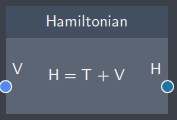# Hamiltonian

## Description

The Hamiltonian node defines the total energy of the system. It is the first building block to get the energy spectrum of a system.## Input

The node has the following input:

• Potential (V): As the Hamiltonian is the sum of the potential and kinetic energy of a system, it requires a potential function defined in the Potential node as its input.

## Content

The content in the node shows what is being calculated. It is the sum of the kinetic and potential energy. The kinetic part $T = \frac{-\hbar^2}{2m} \frac{\partial^2}{\partial x^2}$ is calculated from the spatial dimension $x$, while the Potential ($V$) is taken from the Potential node.

## Output

After the input is provided, the node gives the following output:

• Hamiltonian (H): The Hamiltonian of the defined system.

## Example

In the example below, the Hamiltonan of an infinite well potential is calculated to plot the eigenstates and calculate the spectrum of eigenenergies.